# Exponents and Powers

We can use exponents to rewrite a long multiplication problem: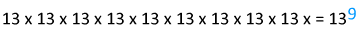There are 9 13s in the problem above, so we rewrite the problem with an exponent of 9.

The exponent is the little number above the 13 and it tells us how many times we are multiplying the 13 by itself.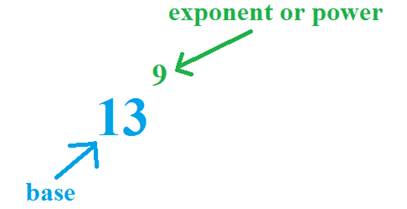There are two special exponents that you may have heard before:These two are very commonly used in geometry. A squared value is often used to represent area. A cubed value is often used to represent volume.

All other exponents are generally read by saying "to the ____ power"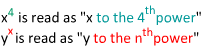Exponents can be used with any real number or variable representing a real number.

ExamplesNotice that the part that repeats always becomes the base. The number of times it repeats is the exponent or the power.

Solving with exponents:

There are a couple of important rules to remember:

Rule 1: Anything to the zero power is equal to 1.So here are some examples using 3 as the base.

Rule 2: Anything to the first power is equal to the base.

Remember that the exponent tells us how many of the base that we have.

Look at the pattern below:A common mistake is to multiply the base and exponent together. Instead, write it out and take the time to solve.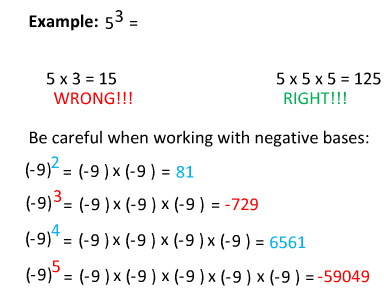You should see that:

Even powers result in positive values.

Odd powers result in negative values.

The placement of the parentheses also changes the answer.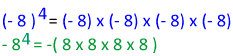(-8) 4 tells us to multiply -8 times itself 4 times.

However, -84 tells us to multiply 8 by itself 4 times and then make it negative. This is because -84 is the same as -(84). The exponent comes before the multiplication in the order of operations. You can add in the parentheses if it helps you to solve the question.

Here is another example. Notice how adding in parentheses changes the problem.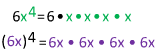Conclusions:

Exponents are a useful tool. They are used to show repeated multiplication. The number of times a number is multiplied by itself is the exponent. However, when we rewrite an expression using exponents, we need to be careful of the placement of negatives and parentheses.

Math
Fractions
Factors Exponents
The Laws of Exponents
Integers as Exponents
Evaluate Exponents
Evaluate integers with exponents
Positive and Negative Integer Exponents
Rational (Fractional) Exponents
Rational (Fractional) Exponents
Zero Exponents